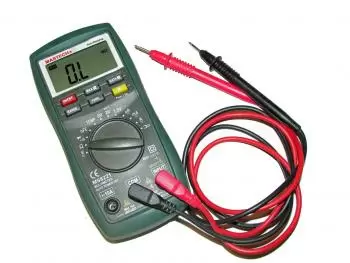# Nominal Voltage - Concept, Definition and ExampleThe rated voltage is the specific potential difference for which an electrical installation or equipment is designed. It is also called nominal voltage.

The nominal voltage of an electrical appliance is the voltage that must not exceed in normal operation. The nominal adjective is because this tension usually serves to characterize the apparatus.

The nominal value indicates the theoretical or ideal value of anything that can be quantified. It is not the actual value. The actual value is obtained in a measurement.

Being a nominal value implies that the voltage can vary for different circumstances.

Depending on the type of electrical system, the rated voltage can be given in:

The nominal tension of a cable is the tension for which the cable has been built and defines the electrical tests.

## What Is the Difference in Electrical Potential?

The electrical potential difference is the energy required to separate electrical charges of opposite sign. It is also known as voltage. The tension increases the more they grow:

• The total charge.

• The distance.

• Intercurrent resistance forces between loads.

Voltage is the difference between the electric potential of two points in space. It is the difference between the potential electrical energy that a charge has at the two points divided by the value of the charge itself. This difference is due to the presence of an electric field.

The difference in electrical potential is measured with a voltmeter. Within the International System of Units, the unit of measurement for the difference in electrical potential is the volt (V).

## Rated Voltage with an Example

To better understand these concepts we compare an electrical circuit with a hydraulic circuit.

The potential difference would correspond to the pressure difference generated in a closed tube filled with liquid with the ends placed at different heights. The difference between two points in the electrical circuit corresponds to the pressure difference between two points in the hydraulic circuit.

Comparing concepts:

• The potential difference between the poles of the electric generator would be the height difference of the tanks of the hydraulic circuit.

• The dissipation of electrical energy would be the consequence of the friction of the liquid with the inner walls of the tube.

• The intensity of the current would correspond to the flow rate of the liquid in the tube.

• The rated voltage would be the pressure difference with which the water circuit has been designed.

The water flow can do work by sliding from a high-pressure point to a low-pressure point. Similarly, electrons moving between two points with different potentials constitute an electric current.

This current can power, for example, an electric motor or in any case provide power in other ways.

Author:

Published: May 13, 2015
Last review: June 3, 2020